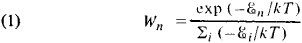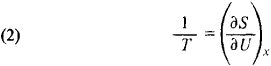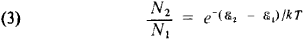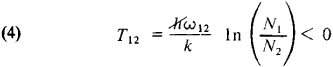# Negative Temperature

Also found in: Wikipedia.

## Negative temperature

The property of a thermodynamical system which satisfies certain conditions and whose thermodynamically defined absolute temperature is negative. The essential requirements for a thermodynamical system to be capable of negative temperature are: (1) the elements of the thermodynamical system must be in thermodynamical equilibrium among themselves in order for the system to be described by a temperature at all; (2) there must be an upper limit to the possible energy of the allowed states of the system; and (3) the system must be thermally isolated from all systems which do not satisfy both requirements (1) and (2); that is, the internal thermal equilibrium time among the elements of the system must be short compared to the time during which appreciable energy is lost to or gained from other systems.

The second condition must be satisfied if negative temperatures are to be achieved with a finite energy. Most systems do not satisfy this condition; for example, there is no upper limit to the possible kinetic energy of a gas molecule. Systems of interacting nuclear spins, however, have the characteristic that under suitable circumstances they can satisfy all three of the conditions, in which case the nuclear spin system can be at negative absolute temperature. See Kinetic theory of matter, Statistical mechanics

The transition between positive and negative temperatures is through infinite temperature, not absolute zero; negative absolute temperatures should therefore not be thought of as colder than absolute zero, but as hotter than infinite temperature. See Absolute zero, Temperature

McGraw-Hill Concise Encyclopedia of Physics. © 2002 by The McGraw-Hill Companies, Inc.
The following article is from The Great Soviet Encyclopedia (1979). It might be outdated or ideologically biased.

## Negative Temperature

an effective quantity introduced for the description of nonequilibrium states of quantum systems. A system is in a negative temperature state when high energy levels are more populated than low energy levels. The probability for a system in an equilibrium state to be on the level ℰn is determined by the equation.Here, £i, are the energy levels of the system, k is the Boltzmann constant, and T is the absolute temperature, which characterizes the mean energy of the equilibrium system U = Σ Wn£n Equation (1) indicates that the low energy levels are more populated by particles than the high energy levels. If external influences cause the system to enter a nonequilibrium state characterized by a greater population of the higher levels than the lower levels, it is formally possible to make use of equation (1) by setting T < 0. The concept of negtive temperature is applicable only to quantum systems that contain a finite number of levels.

The absolute temperature T is defined in thermodynamics through its reciprocal l/T, which is equal to the derivative of the entropy S with respect to the mean energy of the system, the remaining parameters x being kept constant:Equation (2) indicates that the negative temperature implies a decrease of entropy with increasing mean energy. Negative temperature, however, is introduced for the description of nonequi librium states, to which the laws of equilibrium thermodynamics are applicable only conditionally.

An example of a system with negative temperature is the system of nuclear spins in a crystal located in a magnetic field when the spins interact very weakly with the thermal lattice vibrations—that is, a system practically isolated from thermal motion. The time required for the attainment of thermal equilibrium of the spins with the lattice is of the order of several tens of minutes. During this time, the system of nuclear spins may be in a state with negative temperature, a state it entered under external influences.

In a narrower sense, negative temperature is a characteristic of the degree of inversion of the populations of two selected energy levels in a quantum system. In the case of thermodynamic equilibrium, the relation between the populations N1 and N2 of the levels £1 and £21 < £2), that is, the mean numbers of particles in these states, is given by the Boltzmann equation:where T is the absolute temperature of the substance. Equation (3) implies that N2 < N1. Let us suppose the equilibrium of the system is disturbed, for example, by irradiation of the system with monochromatic electromagnetic radiation, whose frequency is close to the frequency of the transition between the levels ω21 = (£2— £1) ℏ and is different from the frequencies of other transitions. It is then possible to attain a state in which the population of the upper level is greater than that of the lower level: N2 > N1. If the Boltzmann equation is conditionally applied to such a nonequilibrium state, negative temperature can be introduced with respect to the pair of energy levels £1 and £2 in accordance with the equationDespite its formal character, this definition is convenient in a number of cases. For example, it permits fluctuations in equilibrium and nonequilibrium systems with negative temperature to be described by analogous equations. The concept of negative temperature is used in quantum electronics for a convenient description of the processes of amplification and generation in mediums with population inversion.

D. N. ZUBAREV

## negative temperature

[′neg·əd·iv ′tem·prə·chər]
(thermodynamics)
The property of a thermally isolated thermodynamic system whose elements are in thermodynamic equilibrium among themselves, whose allowed states have an upper limit on their possible energies, and whose high-energy states are more occupied than the low-energy ones.
McGraw-Hill Dictionary of Scientific & Technical Terms, 6E, Copyright © 2003 by The McGraw-Hill Companies, Inc.
References in periodicals archive ?
The Good-Ark GCR diode has a negative temperature coefficient in order to protect the LED at high temperatures.
A negative temperature coefficient (NTC) thermistor can be configured with the AUXIN analog inputs to accurately monitor module or battery-cell temperature.
Positive temperature coefficient means an insecticide is more toxic at high temperatures while insecticides with negative temperature coefficient are more toxic at low temperatures (Glunt et al., 2013).
Negative temperature and chilly winds don't make a good combination.
The negative temperature bias in northern Atlantic and Europe may also possibly be linked to improper simulation of Gulf Stream, which carries warm water erroneously towards the Canadian Archipelago with consequent impacts on the simulated North American climate.
manufactures negative temperature coefficient thermistors as well as thermistor probes and assemblies with product lines that include thin film platinum resistance temperature detectors and RTD assemblies.
Luckily, reactors also have an inherent ability to self-correct via negative temperature reactivity feedback; reactor power automatically decreases as core temperature increases, and vice versa.
Although reactor trip with control rod drop is equipped, the trip of helium circulator is enough for trip reactor, because the negative temperature feedback provides enough reactivity compensation even for large reactivity insertion accident, taking into account the following fact of residual decay removal mechanism.
A negative slope directly revealing a negative Temperature Coefficient of Resistance (TCR) and high resistivity values of this ultrathin TiN layer in this MOSCAP structure is linked with the metal-semimetal transition phenomena observed in TiN Atomic Layer Deposition experiments .
Use the 16 code forms to express, the first five bit of binary is the sign bit, and 12-bit data is stored in DS18B20 RAM, and take different measures of the temperature conversion method on positive temperature and negative temperature. If the temperature is positive, then the value got is multiplied by 0.0625 to determine the actual temperature; if the temperature is negative, then take back and add 1, and then multiplied by 0.0625 to obtain the actual temperature.

Site: Follow: Share:
Open / Close